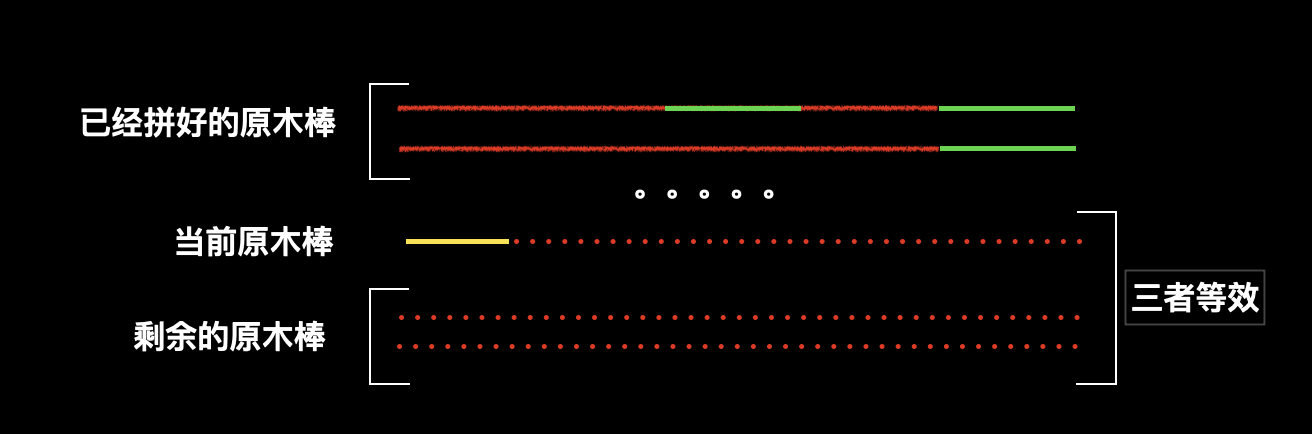V1

2022/08/27阅读：26主题：凝夜紫

# AcWing 165. 小猫爬山

1≤N≤18,
1≤Ci≤W≤108


5 1996
1
2
1994
12
29
**输出样例**
2


## 题目分析

1. u：当前安排的是第几只猫咪，如果当前所有小猫都运送完毕，说明找到了一个方案。
2. k：现有的缆车数量，我们对于每只小猫都枚举这些缆车，看是否可以放进去。
3. sum[]：全局变量，存储每个缆车中现有的猫咪总重量。

1. 把猫咪按照重量排序，先安排比较重的猫咪，占用缆车的重量就多，缆车的剩余重量少，那么后续的可选方案也就更少，这意味着决策树的分支更少。

2. 搜索的时候发现当前的缆车数量 k 已经大于或者等于最佳方案的数量，就可以立即停止搜索了。

## Code

#include <iostream>#include <cstring>#include <algorithm>using namespace std;const int N = 20;int cats[N], sum[N];int n, m;int res = N;void dfs(int u, int k) {    if (k >= res) return ;    if (u == n) {        res = k;        return ;    }    for (int i = 0; i < k; i ++ ) {        if (sum[i] + cats[u] <= m) {            sum[i] += cats[u];            dfs(u + 1, k);            sum[i] -= cats[u];        }    }    sum[k] = cats[u];    dfs(u + 1, k + 1);    sum[k] = 0;}int main() {    cin >> n >> m;    for (int i = 0; i < n; i ++ ) cin >> cats[i];    sort(cats, cats + n);    reverse(cats, cats + n);    dfs(0, 0);    cout << res << endl;    return 0;}

# AcWing 166. 数独

4.....8.5.3..........7......2.....6.....8.4......1.......6.3.7.5..2.....1.4......
......52..8.4......3...9...5.1...6..2..7........3.....6...1..........7.4.......3.
end


417369825632158947958724316825437169791586432346912758289643571573291684164875293
416837529982465371735129468571298643293746185864351297647913852359682714128574936


## 题目分析

1. 优化搜索的顺序。最常用的剪枝之一，本题中，虽然理论上可以选择任意位置填数字，但是考虑后续分支的数量，一定是选择当前可选数字最少的位置，这样留给后续的分支也会更少。

2. 选择了一个位置放数字后就可以直接进入下一层，而不需要把其他位置都选择上。我们可以通过回溯，来枚举出所有的方案。

3. 需要频繁的判断某个数字是否可以放到某个格子，为了加速判断，我们可以使用位运算来进行优化。因为总共有九位数字，所以我们可以用一个九位的二进制数来存储，某个数字有没有被使用过，例如：101011000，即可表示24789这几个数字已经被使用。

## Code

1. 需要每次搜索，需要找到当前可选数字最少的格子。如何判断某个格子的可选数字呢？可以把三个二进制数字取并集，也就是做&操作。这样结果为 1 的二进制位就是可以选的数字。

2. 我们可以用 lowbit 操作统计某个二进制数字有多少个 1。

3. 为了快速找到某个二进制位的 1 的数量，我们可以先预处理出一个数组来存储每个数字 1 的数量。

4. 为了快速找到最后一个 1 的位置，也就是需要操作的格子，我们可以使用一个数组来存放每个二进制数对应的最后一个 1 的位置。

#include <iostream>#include <cstring>#include <algorithm>using namespace std;const int N = 9;// cell 存储的是当前格子在哪个 以3*3为单位的小矩阵中int row[N], col[N], cell;char str;// ones[i]表示i中有几个1 用来快速找到1 最少的那个格子// map[i] 表示最后的一个1 后面有几个0 也就是i的最后一个1在第几位。// 比如lowbit返回二进制(1000) 那么结果就是8 而map则存储map = 3int ones[1 << N], map[1 << N];// inline 可以直接把函数体直接放到调用的部分 省去调用函数的开销inline int lowbit(int x) { // 返回最后一个1    return x & -x;}// 初始化 行列格 的选择状态 为全部可选。void init() {    for (int i = 0; i < N; i ++ ) row[i] = col[i] = (1 << N) - 1;    for (int i = 0; i < 3; i ++ )        for (int j = 0; j < 3; j ++ )            cell[i][j] = (1 << N) - 1;}// 求可选方案的交集inline int get(int x, int y) {    return row[x] & col[y] & cell[x / 3][y / 3];}bool dfs(int cnt) {    if (!cnt) return true;    // 找出可选方案数最小的格子    int minv = 10;    int x, y;    for (int i = 0; i < N; i ++ )        for (int j = 0; j < N; j ++ ) {            if (str[i * N + j] == '.') { // 如果格子可选                int t = ones[get(i, j)];                if (t < minv) {                    minv = t;                    x = i, y = j;                }            }        }    // cout << x << ' ' << y << endl;    for (int i = get(x, y); i; i -= lowbit(i)) {        int t = map[lowbit(i)];        // 修改状态 把最后一个1变成0        row[x] -= 1 << t;        col[y] -= 1 << t;        cell[x / 3][y / 3] -= 1 << t;        // x * 9 + y 把二维坐标转换成一维中的位置        str[x * 9 + y] = '1' + t;        if (dfs(cnt - 1)) return true;        // 回溯        row[x] += 1 << t;        col[y] += 1 << t;        // 在从一维中的位置 转换到二维坐标        cell[x / 3][y / 3] += 1 << t;        str[x * 9 + y] = '.';    }    return false;}int main() {    for (int i = 0; i < N; i ++ ) map[1 << i] = i;    for (int i = 0; i < 1 << N; i ++ ) {        int s = 0;        for (int j = i; j; j -= lowbit(j)) s ++ ;        ones[i] = s; // i的二进制表示中有s个1    }    while (cin >> str, str != 'e') {        init();        int cnt = 0;        for (int i = 0, k = 0; i < N; i ++ )            for (int j = 0; j < N; j ++, k ++ )                if (str[k] != '.') {                    int t = str[k] - '1';                    str[i * N + j] = t + '1';                    int v = 1 << t;                    row[i] -= v;                    // 2022年8月8日 把col[j]写成了col[i]找了一个半小时                    // 晚23:40终于找到了                    col[j] -= v;                    cell[i / 3][j / 3] -= v;                }                else cnt ++ ; // 没填的格子总共有cnt个        dfs(cnt);        cout << str << endl;    }    return 0;}

# AcWing 167. 木棒

9
5 2 1 5 2 1 5 2 1
4
1 2 3 4
0


6
5


## 题目分析

（我们约定，多跟木棍组成木棒，木棍的长度由题目给出。）

1. 同上题一样，对于搜索顺序，我们搜索更大的木棍，就可以留出更小的空间给后续选择，也就意味着后续分支会更少，所以可以先对所有的木棍排序。

2. 另外一个比较明显的剪枝是：因为木棍已经是有序的，那么如果当前木棍不能拼接在当前木棒上，那么和当前木棍相同长度的也不能拼接到当前木棒上，直接跳过即可。

3. 还有一个不明显的剪枝是：当前组合失败时，当前木棍是第一根组成木棒的木棍，说明当前方案直接失败，因为后续的空木棒也是没有任何木棍组成的，而当前木棍作为这个空木棒的第一根组成木棍失败，再其他空木棒作为第一根组成木棍也会失败。1. 另外一个不明显的剪枝是：当前组合失败时，当前木棍是最后一根组成木棒的木棍，同样说明该方案直接失败。

该结论可以使用反证法证明：假设当前处于最后一根的木棍拼在当前木棒中，我们称为原状态，而原状态失败，但是把该木棒拼在后面又可以成功了，那说明原状态中本来拼在后面的一定有一段木棍组合与当前的最后一根是完全相等的长度，那么把他们调换一下也是可以的，那样原状态应该也是成功的，但是在假设中原状态是失败的。


## Code

#include <iostream>#include <cstring>#include <algorithm>using namespace std;const int N = 70;int w[N], sum, length; // sum所有木棒总长 length单根木棒长度bool st[N];int n;// 第u个木棒 当前木棒的长度为s 组成这根木棒的木棍起始下标为startbool dfs(int u, int s, int start) {    // u个长度为len的等长木棒 总和等于 所有木棒长度总和 则找到了方案    if (u * length == sum) return true;    // 当前木棒的长度为length 则新开一个木棒。    if (s == length) return dfs(u + 1, 0, 0);    for (int i = start; i < n; i ++ ) {        if (st[i] ) continue;        // 当前木棍不行 就换后面小的。        if (s + w[i] > length) continue;        st[i] = true;        if (dfs(u, s + w[i], i + 1)) return true;        st[i] = false;        // 如果木棒的第一根木棍失败， 则后面的也一定失败        // 如果当前木棒放在最后一个失败，则当前方案也一定失败        if (!s) return false;        if (s + w[i] == length) return false;        // 剪枝 当前后两根木棍 长度相同的话 前一根木棍不能放进木棒        // 那么后一根也不能放 所以我们可以跳过相同长度的木棍。        int j = i;        while (j < n and w[j] == w[i]) j ++ ;        i = j - 1;    }    return false;}int main() {    while (cin >> n, n) {        memset(st, 0, sizeof st);        sum = 0;        for (int i = 0; i < n; i ++ ) {            cin >> w[i];            sum += w[i];        }        // 把木棍从大到小排列 这样每次枚举大的 剩下的空间小 分支少        sort(w, w + n);        reverse(w, w + n);        length = 1;        while (true) {            // 剪枝1            if (sum % length == 0 and dfs(0, 0, 0)) {                cout << length << endl;                break;            }            length ++ ;        }    }    return 0;}

# AcWing 168. 生日蛋糕

7 月 17 日是 Mr.W 的生日，ACM-THU 为此要制作一个体积为 Nπ 的 M 层生日蛋糕，每层都是一个圆柱体。

1≤N≤10000,
1≤M≤20


100
2


68


## 题目分析

1. 外表面积 Q 分为两部分，一部分是最下层圆柱的圆形面积，另一部分是每个圆柱体展开成长方形之后的侧面积之和。也就是： 而题目中给出 ，所以可以同时两面约掉 ，于是得到
2. 给定总体积 N，和总层数 M，求最小的 S，也就是求使 S 最小的 R 和 H。
3. 总体积的计算是圆形的面积乘以高度也就是：
4. 从最上层往下，每一层的半径和高度都是严格递增的。

1. dep: 当前搜索的是哪一层;
2. s，v: 当前总共使用了的表面积和体积;
3. h，r：记录每层所使用的高度和半径;

1. 上下界剪枝。根据题目信息 4，如果每层的半径和高度至少是 1，那么当前 dep 层的半径和高度就至少要大于等于 dep，并且小于等于

除此之外，还要考虑到体积的影响，如果当前体积是 v，而总体积是 V，那么所剩下的可用体积就是 ，所以当前层最大的体积不能超过这个值，也就是 ，而其中 的最小取值是1，所以 的最小取值就是 ，所以 的最小取值就是

综上所述，我们可以得到每层的 R 和 H 的取值：
,

2. 优化搜索顺序，我们使用倒序枚举，因为越靠近底层，则占用的面积和体积越大，留给上层的也就越小，决策树的分支也越少。

同理，R 和 H 的枚举也要从大到小，并且因为 R 对表面积的影响比较大，所以先枚举 R。

3. 可行性剪枝。我们可以预处理出来每层最小的可使用面积mins[]和体积minv[]，这样，如果当前的已使用体积加上当前层的最小体积超过了总体积 V，就可以剪枝；如果当前已使用的表面积加上当前层的表面积超过了答案（也就是当前最优选择的表面积），也可以直接剪枝。

4. 最优性剪枝
先来看一下当前1~dep层的表面积公式(忽略 )：

再来看一下当前1~dep层的体积公式：

现在来找一下这两个公式之间的关系，我们对 做一个等效变形，将公式中的 2 提到前面，然后除以一个 ，再乘以一个 ，也就是变成这样：

因为R是递减的，所以 ，所以上述公式把后面的 替换成 ，得到

此时，我们神奇的发现，表面积公式 的后半部分就是体积公式

因此，我们就可以得到：

加上下一层的表面积 s，就一定有： （其中 s 为下一层的表面积，在边界条件下可以取到等于，也就是在最后一层时，两面都是 0）。

## Code

#include <cstring>#include <iostream>#include <algorithm>#include <cmath>using namespace std;const int N = 25, INF = 1e9;int V, m;int minv[N], mins[N]; // 每层面积和体积的最小值int R[N], H[N]; // 每层的半径和高度int res = INF;void dfs(int dep, int v, int s) {    // 剪枝3    if (v + minv[dep] > V) return ;    if (s + mins[dep] >= res) return ;    // 剪枝4    if (s + 2 * (V - v) / R[dep + 1] >= res) return ;    if (!dep) {        if (v == V) res = s;        return ;    }    // 枚举当前层的r和h，剪枝1和剪枝2    for (int r = min(R[dep + 1] - 1, (int)sqrt(V - v)); r >= dep; r -- ) {        for (int h = min(H[dep + 1] - 1, (V - v) / r / r); h >= dep; h -- ) {            // cout << r << h << endl;            int t = 0;            // 注意如果是最底层需要把上面的圆形面积也加上            if (dep == m) t = r * r;            R[dep] = r;            H[dep] = h;            // 加上当前体积和当前表面积            dfs(dep - 1, v + r * r * h, s + 2 * r * h + t);        }    }}int main() {    cin >> V >> m;    // 剪枝3初始化    for (int i = 1; i <= m; i ++ ) {        // 每层半径和高度最小值都等于当前层数        minv[i] = minv[i - 1] + i * i * i;        mins[i] = mins[i - 1] + 2 * i * i;    }    // 其中有一步要用到R和H的上一层-1    // 所以我们初始化一个哨兵，方便操作    R[m + 1] = H[m + 1] = INF;    dfs(m, 0, 0);    if (res == INF) res = 0;    cout << res << endl;    return 0;}

# 总结

1. 什么时候可以考虑使用深度优先搜索？

有一个重要的条件，就是数据范围比较小，观察我们两篇文章中的九个题目，数据范围全部在 100 以下。这种数据范围下，我们有可能通过枚举出所有的方案，来找到题目要求的最佳方案。

2. 深度优先搜索的两种常用搜索顺序。

1. 对于物品来枚举组。物品作为递归函数的参数，在递归函数中，尝试把物品放在每个组中。

例如：考虑一堆水果，要把他们分到不同的篮子里面，我们可以考虑每个水果放在哪个篮子里面。

这种情况，一般需要用全局变量记录每个组的内部情况。递归的参数除了记录第几个物品之外，还要记录当前共枚举了多少物品，用来判断递归终止条件。

参考题目：AcWing 165. 小猫爬山

2. 对于组来枚举物品。组作为递归函数的参数，在递归函数内部中，枚举每个物品，尝试把所有的物品都放到当前组中。

例如：考虑一堆水果，要把他们分到不同的篮子里面，我们可以考虑用每个篮子去装所有的苹果

这种情况，一般需要一个判重数组，来记录哪个物品已经被使用，因为对于每一个组，都要枚举所有物品。并且，除了当前组作为函数的参数之外，还要把当前组的状态也作为函数的参数

参考题目：AcWing 167. 木棒

3. 剪枝。深度优先搜索的时间复杂度是指数级别的，所以就算数据范围通常比较小，但是时间复杂度依然会非常大，所以一般都要配合剪枝。

1. 搜索顺序的剪枝。从大往小搜索，给后续留下的可选范围越小，后续的分支就越少，搜索速度越快。

2. 排除等效冗余。如果决策树中当前节点延伸出的几条分支具有等效性，则只需要搜索其中一条剪枝就行。

3. 可行性剪枝。有些问题可以预处理出来一些状态，利用这些状态在搜索的过程中直接剪枝。

4. 最优性剪枝。如果当前花费的代价已经超过了最优解，则直接剪枝。

ENDV1